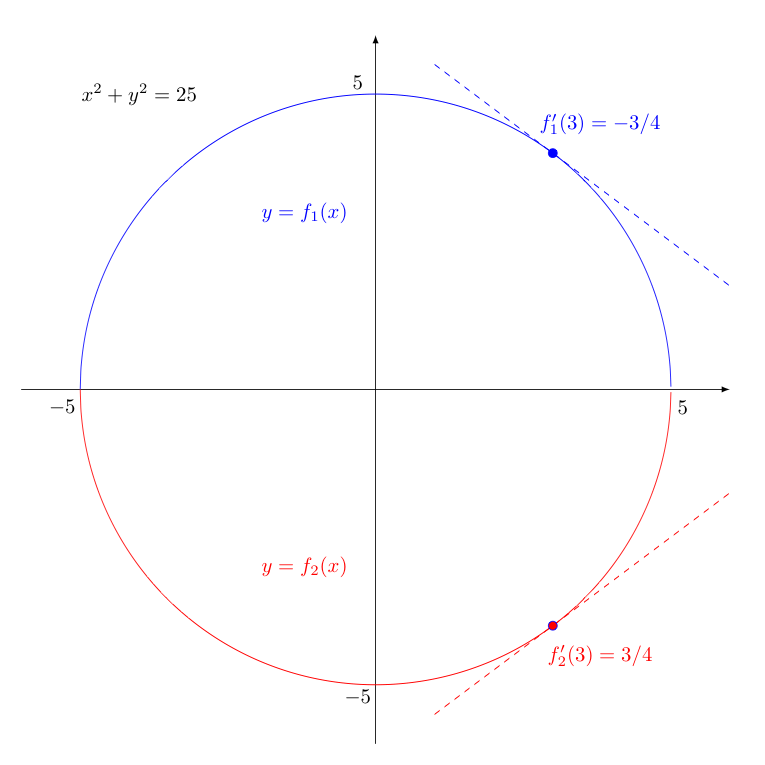## Implicit Differentiation

When one writes a formula for a function, say $f(x) = 3x^2 + 5x + 1$, one is said to have defined the function explicitly. Similarly, when one writes $y = 3x^2 + 5x + 1$, we have explicitly defined $y$ in terms of $x$.

However, for some equations relating $x$ and $y$, like $x^6 - 2x = 3y^6 + y^5 - y^2$, there is no easy way to solve for $y$ explicitly in terms of $x$. Still, there may exist functions $f(x)$ so that if we plug $y=f(x)$ into this equation, it holds true. The possible functions $f(x)$ that have this property are then said to be defined implicitly by the given equation.

With the assumption that a given equation implicitly defines at least one differentiable function, one can find the derivative of one variable in terms of another through a process known as implicit differentiation.

Consider the following:

Suppose we are asked to find $\displaystyle{\frac{dy}{dx}}$ given

$$3x^4 y^2 - 7xy^3 = 4 - 8y$$

Our strategy is to treat the left and right sides of the equation above as functions of $x$ in their own right, and differentiate them both with respect to $x$. As the left and right sides agree, their derivatives should be equal as well. This will give us an equation in terms of an unknown $dy/dx$ for which we may then solve.

Importantly, as we differentiate each side, we treat $y$ as a function of $x$. This requires that whenever we take a derivative of a function of $y$ (e.g., $y^2$, or $y^3$), we must invoke the chain rule and pick up an additional factor of $dy/dx$.

Thus, as an example, just as $\displaystyle{\frac{d}{dx}\left([f(x)]^3\right) = 3[f(x)]^2 \cdot f'(x)}$, we find $\displaystyle{\frac{d}{dx}\left(y^3\right) = 3y^2\frac{dy}{dx}}$.

Differentiating in this way both sides of the equation above results in the following (after applying the product rule twice):

$$\left[ 12x^3 y^2 + 3x^4 \cdot 2y \frac{dy}{dx} \right] - \left[ 7y^3 + 7x \cdot 3y^2 \frac{dy}{dx} \right] = 0 - 8 \frac{dy}{dx}$$

Note how the result is linear in terms of the $dy/dx$. Nicely, if the original equation is in terms of only $x$s and $y$s, this always happens! As such, we can always easily solve for this unknown $dy/dx$, by collecting all of the $dy/dx$ terms on one side, with all other terms on the other. Doing this here produces the following:

$$(6x^4 y - 21 xy^2 + 8) \cdot \frac{dy}{dx} = 7y^3 - 12x^3 y^2$$

Dividing by the factor to the left of $dy/dx$, we then have

$$\frac{dy}{dx} = \frac{7y^3 - 12x^3 y^2}{6x^4y-21xy^2+8}$$

As one can see, the resulting expression for $dy/dx$ does involve $y$ values. This almost always happens -- but it should be no surprise, as the original equation we were given may actually implicitly define multiple functions.

To understand this better, suppose we started instead with $x^2 + y^2 = 25$, whose graph is a circle. The two functions $f_1$ and $f_2$ that are implicitly defined by this equation correspond to the semi-circles on the top and bottom halves of this circle, as shown below. For any given $x$ value (e.g., $x=3$), we thus have two possible tangent lines with two potentially different slopes (and hence, values of $dy/dx$). To find the slope of a tangent line to the curve at some $x$-value, we need to know to which function we hope to be tangent. The $y$-value gives us this information.